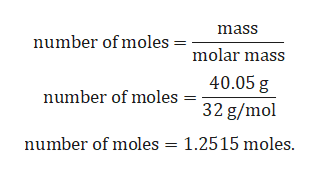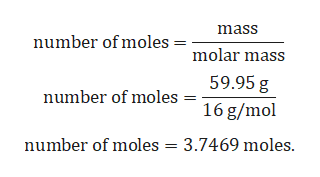# A compound is found to contain 40.05 % sulfur and 59.95 % oxygen by mass.What is the empirical formula for this compound?

Question
769 views

A compound is found to contain 40.05 % sulfur and 59.95 % oxygen by mass.

What is the empirical formula for this compound?

check_circle

Step 1

Given:

Mass percent of sulfur = 40.05 %.

Mass percent of oxygen = 59.95 %.

Molar mass of oxygen = 16 g/mol.

Molar mass of sulfur = 32 g/mol.

Conversion of mass percent to gram:

Since we are having mass percent, it is convenient to use 100 g of the compound as the starting material. Thus, in the 100 g of sample of above compound 40.05 g sulfur and 59.95 g of oxygen.

Step 2

Calculation for number of sulfur:help_outlineImage Transcriptionclosemass number of moles molar mass 40.05 g number of moles 32 g/mol number of moles 1.2515 moles. fullscreen
Step 3

Calculation for numb...help_outlineImage Transcriptionclosemass number of moles molar mass 59.95g number of moles 16 g/mol 3.7469 moles number of moles fullscreen

### Want to see the full answer?

See Solution

#### Want to see this answer and more?

Solutions are written by subject experts who are available 24/7. Questions are typically answered within 1 hour.*

See Solution
*Response times may vary by subject and question.
Tagged in
ScienceChemistry

### General Chemistry